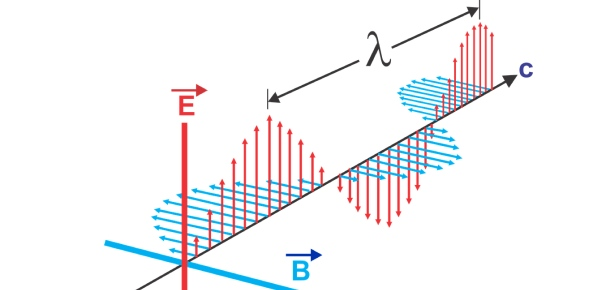# Chapter 22: Electromagnetic Waves

35 Questions | Attempts: 327
ShareSettings• 1.
The basic equations for all electromagnetism were developed by
• A.

Newton.

• B.

Gauss.

• C.

Maxwell.

• D.

Hertz.

• 2.
An electric field is produced by a
• A.

Constant magnetic field.

• B.

Changing magnetic field.

• C.

Either a constant or a changing magnetic field.

• D.

• 3.
A changing electric field will produce a
• A.

Current.

• B.

Gravitational field.

• C.

Magnetic field.

• D.

• 4.
All electromagnetic waves travel through a vacuum at
• A.

The same speed.

• B.

Speeds that are proportional to their frequency.

• C.

Speeds that are inversely proportional to their frequency.

• D.

• 5.
In a vacuum, the velocity of all electromagnetic waves
• A.

Is zero.

• B.

Is nearly 3 * 10^8 m/s.

• C.

Depends on the frequency.

• D.

Depends on their amplitude.

• 6.
Electromagnetic waves can travel through
• A.

Glass.

• B.

Iron.

• C.

Water.

• D.

• 7.
The strength of both the electric and magnetic fields in the radiation field are found to decrease with distance as
• A.

1/r.

• B.

1/r^2.

• C.

1/r^3.

• D.

1/r^4.

• 8.
The E and B fields in electromagnetic waves are oriented
• A.

Parallel to the wave's direction of travel, as well as to each other.

• B.

Parallel to the waves direction of travel, and perpendicular to each other.

• C.

Perpendicular to the wave's direction of travel, and parallel to each other.

• D.

Perpendicular to the wave's direction of travel, and also to each other.

• 9.
An electromagnetic wave is traveling to the east. At one instant at a given point its E vector points straight up. What is the direction of its B vector?
• A.

North

• B.

Down

• C.

East

• D.

South

• 10.
An electromagnetic wave is radiated by a straight wire antenna that is oriented vertically. What should be the orientation of a straight wire receiving antenna? It should be placed
• A.

Vertically.

• B.

Horizontally and in a direction parallel to the wave's direction of motion.

• C.

Horizontally and in a direction perpendicular to the wave's direction of motion.

• D.

• 11.
Electromagnetic waves are
• A.

Longitudinal.

• B.

Transverse.

• C.

Both longitudinal and transverse.

• D.

Neither longitudinal or transverse.

• 12.
Of the following, which is not electromagnetic in nature?
• A.

Microwaves

• B.

Gamma rays

• C.

Sound waves

• D.

• 13.
Visible light ranges in wavelength from
• A.

400 μm to 750 μm.

• B.

400 nm to 750 nm.

• C.

500 μm to 850 μm.

• D.

500 nm to 850 nm.

• 14.
The energy an electromagnetic wave transports per unit time per unit area is the
• A.

Energy density.

• B.

Power.

• C.

Intensity.

• D.

• 15.
The force per unit area exerted by an electromagnetic wave is called the
• A.

Energy density.

• B.

Power.

• C.

Intensity.

• D.

• 16.
Which of the following correctly lists electromagnetic waves in order from longest to shortest wavelength?
• A.

Gamma rays, ultraviolet, infrared, microwaves

• B.

Microwaves, ultraviolet, visible light, gamma rays

• C.

Radio waves, infrared, gamma rays, ultraviolet

• D.

Television, infrared, visible light, X-rays

• 17.
If the electric field in an EM wave has a peak value of 2.0 V/m, what is the peak value of the magnetic field?
• A.

6.7 nT

• B.

2.0 T

• C.

3.0 * 10^8 T

• D.

• 18.
What is the wavelength of light waves if their frequency is 5.0 * 10^14 Hz?
• A.

0.60 m

• B.

6.0 mm

• C.

0.060 mm

• D.

0.60 μm

• 19.
What is the wavelength of a 92.9-MHz radio wave?
• A.

32 mm

• B.

32 cm

• C.

3.2 m

• D.

32 m

• 20.
What is the wavelength of a radio wave signal transmitted at a frequency of 7.2 MHz?
• A.

42 m

• B.

4.2 m

• C.

29 m

• D.

0.024 m

• 21.
What is the wavelength for the carrier wave in the station WBRN 1460?
• A.

2.05 m

• B.

20.5 m

• C.

205 m

• D.

2.05 km

• 22.
What is the frequency of 20 mm microwaves?
• A.

100 MHz

• B.

400 MHz

• C.

15 GHz

• D.

73 GHz

• 23.
How far does light travel in 1.0 μs?
• A.

3.0 * 10^14 m

• B.

0.30 km

• C.

3.0 m

• D.

30 cm

• 24.
How long does it take light to travel 1.0 m?
• A.

3.3 ns

• B.

3.3 μs

• C.

3.3 ms

• D.

3.3 s

• 25.
How long does it take the signal from a local radio station to travel 20 miles?
• A.

2.8 s

• B.

108 ms

• C.

38 ms

• D.

108 ms

## Related TopicsBack to top
×

Wait!
Here's an interesting quiz for you.